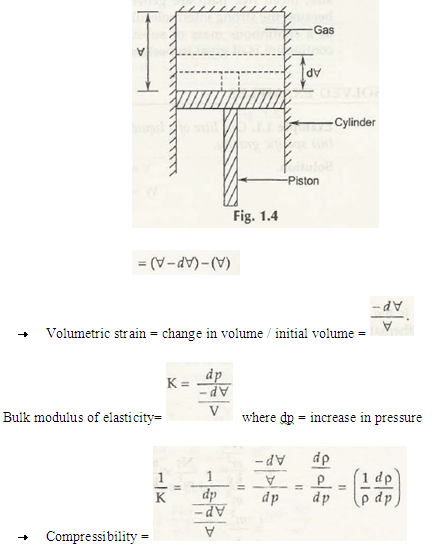In fluid dynamics, compressibility is one of the major factors that determine the nature of fluid. This measurement is necessary to understand the response for pressure change during compression on the gases.It is denoted by (1/ K).

Let us consider initial volume of gas (before compression) be V

Final volume of gas (after compression) V – dV as shown in Fig. 1.4So, Change in volume of gas will be-

(V – dV)– V = – dV

Volumetric Strain

Volumetric strain of fluid can be defined as the ratio of change in volume to the initial volume. Mathematically, it can be written as-

Volumetric Strain = – dV/ V

Bulk modulus of elasticity

It can be defined as the ratio of change in pressure to volumetric strain. It is denote by K.

Mathematically,

K = dp/((– dV/ V))

Or,
1/K = 1/(dp/((– dV/ V)))

1/K = ((– dV/ V))/dp

Or,

1/K = (( dρ/ ρ))/dp

Hence,

1/K = dρ/(ρ dp)

Note:

As compare to liquids, compressibility of gases is high.

Links of Next Mechanical Engineering Topics:-### Customer Reviews

My Homework Help
Rated 5.0 out of 5 based on 510 customer reviews at